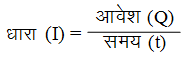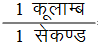# Physics

### Chapter : 1. Electricity

#### Electric Current (Charge In Motion)

Definition : The quantity of electric charge flowing through cross section of a given conductor in one second is called current.
Thus, if Q is the charge which flows through a conductor in time t, then the current (I) is given byThe electric current (or current) is a scalar quantity.
Unit of current
The SI unit of charge (Q) is coulomb (C), and that of time (t) is second (s). So,
SI unit of current= 1 Ampere
The unit coulomb per second (Cs–1) is called ampere (A)
Direction of Electric Current :
The direction of flow of the positive charge taken as conventional direction of the electric current.
When we consider the flow of electric current in an ordinary conductor, such as a copper wire, the direction of current is taken as opposite to the direction of the flow of electrons.